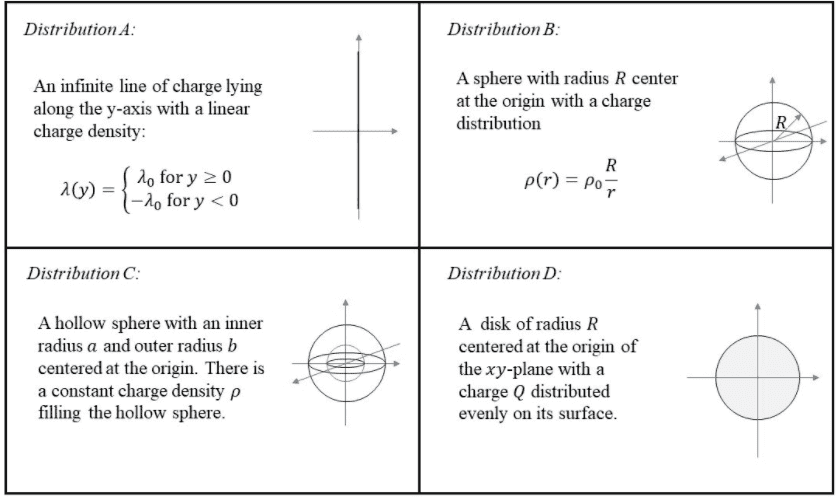# Determining electric field using gauss's law--different distributions

Homework Statement:
We are supposed to determine which (if any) of the distributions will need to/could use Gauss's law to determine the electric field
Relevant Equations:
flux = Q/electric constant = EAThese are the 4 distributions shown, and I have to determine which two distributions (or none at all) can use Gauss's law to determine the electric field.

So electric flux = EA = Q/electric constant.

Since all of them have charges, I could do something like Q/(A*electric constant) to get the electric field—that's where I'm confused, because I think I could actually use Gauss's law on all four of them. The only other way I can think of is that Gauss's law is applied to surfaces so that probably excludes lines (A) and disks (D), which do not really have surface area.
Am I right in thinking so? Is there a better / correct way to get to the answer?

Last edited:

## Answers and Replies

PeroK
Science Advisor
Homework Helper
Gold Member
2020 Award
You perhaps need to think about how you would practically use Gauss's law to determine the electric field.

Hint: think about symmetry.

@PeroK
Hmm. Distribution A could potentially be viewed as line of charge + imaginary cylinder to get some electric field, but the charges aren't symmetric and hence will not be able to use gauss's law (?).
I'm not sure where else it's not symmetric though.

PeroK
Science Advisor
Homework Helper
Gold Member
2020 Award
@PeroK
Hmm. Distribution A could potentially be viewed as line of charge + imaginary cylinder to get some electric field, but the charges aren't symmetric and hence will not be able to use gauss's law (?).
I'm not sure where else it's not symmetric though.
The trick to use Gauss's law effectively is to have a surface across which the electric flux must be constant.

Your intuition is right that A and D are problematic and B and C are better. Can you explain more clearly how you do things in cases B and C?